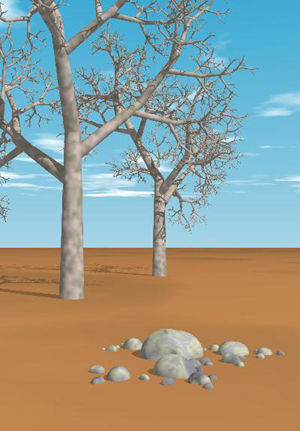Fractal Trees

Fractal trees an plants are among the easiest of fractal objects to understand. They are based on the idea of self-similarity. As can be seen from the example of a fractal tree belowThis tree clearly shows the idea of self-similarity. Each of the branches is a smaller version of the main trunk of the tree. The main idea in creating fractal trees or plants is to have a base object and to then create smaller, similar objects protruding from that initial object. The angle, length and other features of these "children" can be randomized for a more realistic look. This method is a recursive method, meaning that it continues for each child down to a finite number of steps. At the last iteration of the tree or plant you can draw a leaf of some type depending on the nature of the plant or tree that you are trying to simulate. This idea can also be applied to the 3rd dimension by allowing children to be angled in the z-plane as well as in the xy-plane.

Here is some psuedo-code on how to make a tree similar to the one in the picture below:```DrawTree(n, direction, length)

if n > 0 do

DrawTrunk(direction, length)

DrawTree(n-1, 3DRandomAngle(direction), length*Factor(n))

DrawTree(n-1, direction + random % 10, length*Factor(n))

DrawTree(n-1, 3DRandomAngle(direction), length*Factor(n))

else

DrawLeaf()

end if

end DrawTree```

Here are a few examples of fractal trees in action